Next: Nuclear Magnetic Resonance Up: Time-Dependent Perturbation Theory Previous: General Analysis

# Two-State System

Consider a system in which the time-independent Hamiltonian possesses two eigenstates, denoted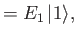(8.13)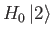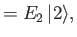(8.14)

where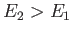. Suppose, for the sake of simplicity, that the diagonal matrix elements of the interaction Hamiltonian,, are zero: that is,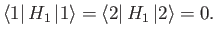(8.15)

The off-diagonal matrix elements are assumed to oscillate sinusoidally at some angular frequency:(8.16)

whereandare real. Note that it is only the off-diagonal matrix elements that give rise to the effect in which we are primarily interested--namely, transitions between states 1 and 2. (See Exercise 1.)

For a two-state system, Equation (8.11) reduces to(8.17)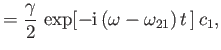(8.18)

where, and it is assumed that. Equations (8.18) and (8.19) can be combined to give a second-order differential equation for the time variation of the amplitude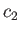: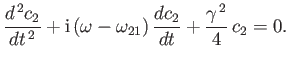(8.19)

Once we have solved for, we can use Equation (8.19) to obtain the amplitude. Let us look for a solution in which the system is certain to be in state 1 at time. Thus, our initial conditions areand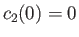. It is easily demonstrated that the appropriate solutions are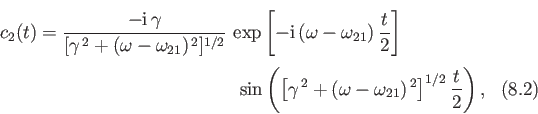andThe probability of finding the system in state 1 at timeis simply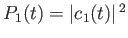. Likewise, the probability of finding the system in state 2 at timeis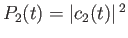. It follows that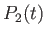(8.20)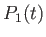(8.21)

Equation (8.23) is generally known as the Rabi formula .

Equation (8.23) exhibits all the features of a classic resonance . At resonance, when the oscillation frequency of the perturbation,, matches the so-called Rabi frequency ,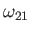, we find that(8.22)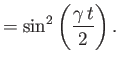(8.23)

According to the previous result, the system starts off atin state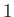. After a time interval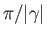, it is certain to be in state 2. After a further time interval, it is certain to be in state 1, and so on. In other words, the system periodically flip-flops between states 1 and 2 under the influence of the time-dependent perturbation. This implies that the system alternatively absorbs energy from, and emits energy to, the source of the perturbation. The absorption-emission cycle also take place away from the resonance, when. However, the amplitude of oscillation of the coefficientis reduced. This means that the maximum value ofis no longer unity, nor is the minimum value ofzero. In fact, if we plot the maximum value ofas a function of the applied frequency,, then we obtain a resonance curve whose maximum (unity) lies at the resonance, and whose full-width half-maximum (in angular frequency) is. Thus, if the applied frequency differs from the resonant frequency by substantially more than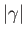then the probability of the system making a transition from state 1 to state 2 is very small (i.e.,). In other words, the time-dependent perturbation is only effective at causing transitions between states 1 and 2 if its angular frequency of oscillation lies in the approximate range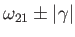. Clearly, the weaker the perturbation (i.e., the smallerbecomes), the narrower the resonance.Next: Nuclear Magnetic Resonance Up: Time-Dependent Perturbation Theory Previous: General Analysis
Richard Fitzpatrick 2016-01-22